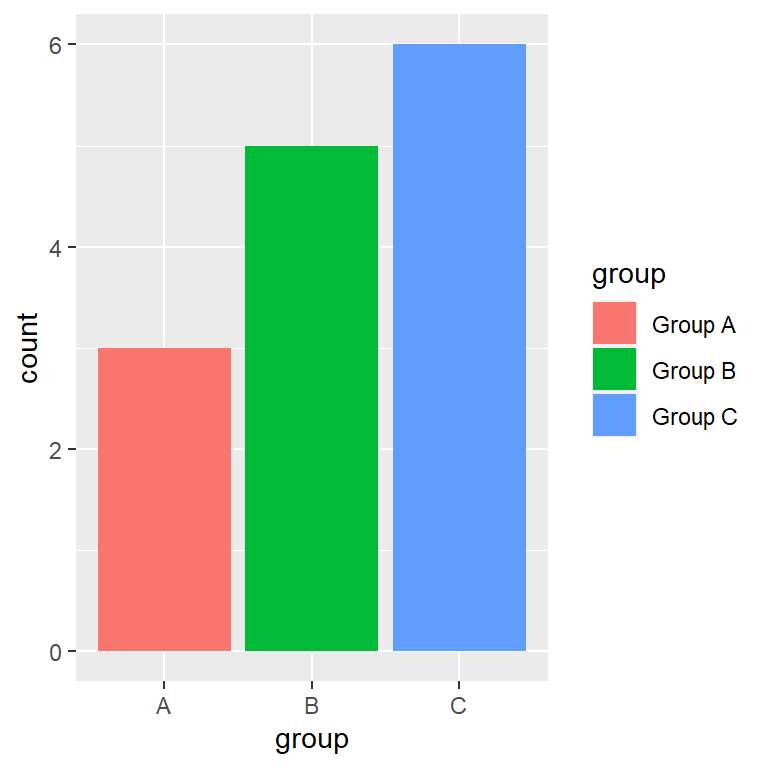# Bar plot in ggplot2 with geom_bar and geom_col

## Sample data sets

When you want to create a bar plot in ggplot2 you might have two different types of data sets: when a variable represents the categories and other the count for each category and when you have all the occurrences of a categorical variable, so you want to count how many occurrences exist for each group. In this tutorial we will show you how to deal with both types of data.

Data with category and count

``````df <- data.frame(group = c("A", "B", "C"),
count = c(3, 5, 6))``````

Only categorical data

``````df2 <- data.frame(cat = c("A", "A", "A",
"B", "B", "B", "B", "B",
"C", "C", "C", "C", "C", "C"))``````

## Bar chart with `geom_bar`

geom_bar

The main function for creating bar plots or bar charts in ggplot2 is `geom_bar`. By default, this function counts the number of occurrences for each level of a categorical variable.

``````# install.packages("ggplot2")
library(ggplot2)

ggplot(df2, aes(x = cat)) +
geom_bar()``````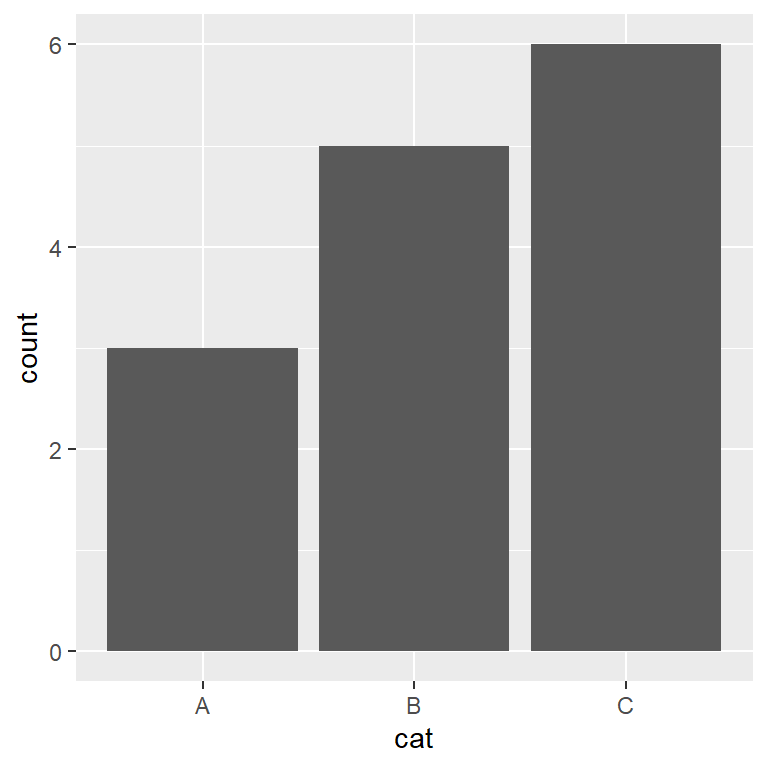geom_bar with stat “identity”

Nonetheless, if your data set already contains the count for each group, as in our sample data set named `df`, you will need to pass `stat = "identity"` inside `geom_bar`.

``````# install.packages("ggplot2")
library(ggplot2)

ggplot(df, aes(x = group, y = count)) +
geom_bar(stat = "identity")``````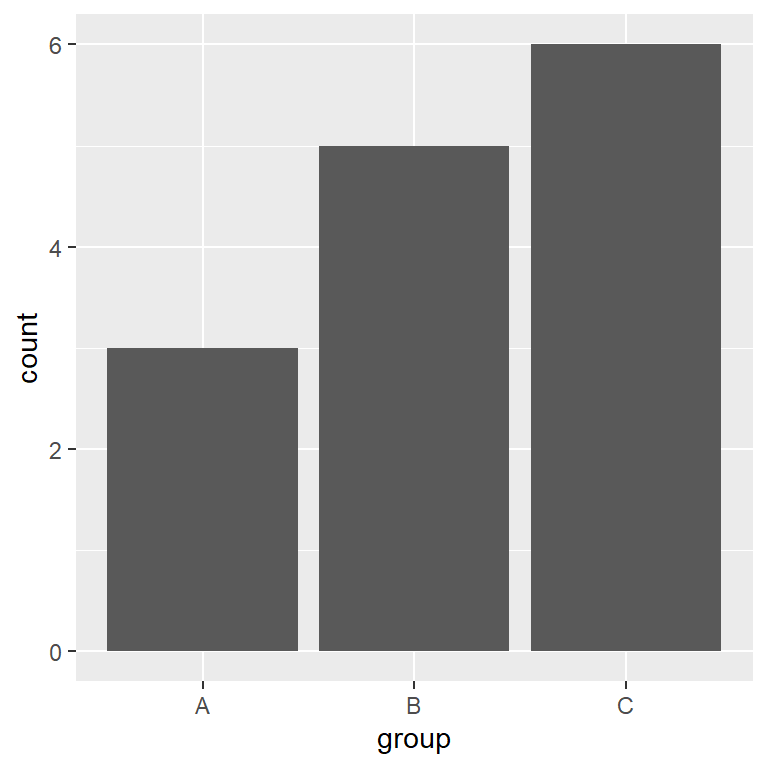## Bar chart with `geom_col`geom_col

`geom_col` is the same as `geom_bar(stat = "identity")`, so if your data contains groups and the count for each group you can just use this function instead.

``````# install.packages("ggplot2")
library(ggplot2)

ggplot(df, aes(x = group, y = count)) +
geom_col()``````

## Horizontal bar plot

There are two ways to create a horizontal bar plot: using the `coord_flip` function to flip the axes or passing the categorical variable to the `y` argument of `aes`.

Option 1: using `coord_flip`

``````# install.packages("ggplot2")
library(ggplot2)

ggplot(df, aes(x = group, y = count)) +
geom_bar(stat = "identity") +
coord_flip()``````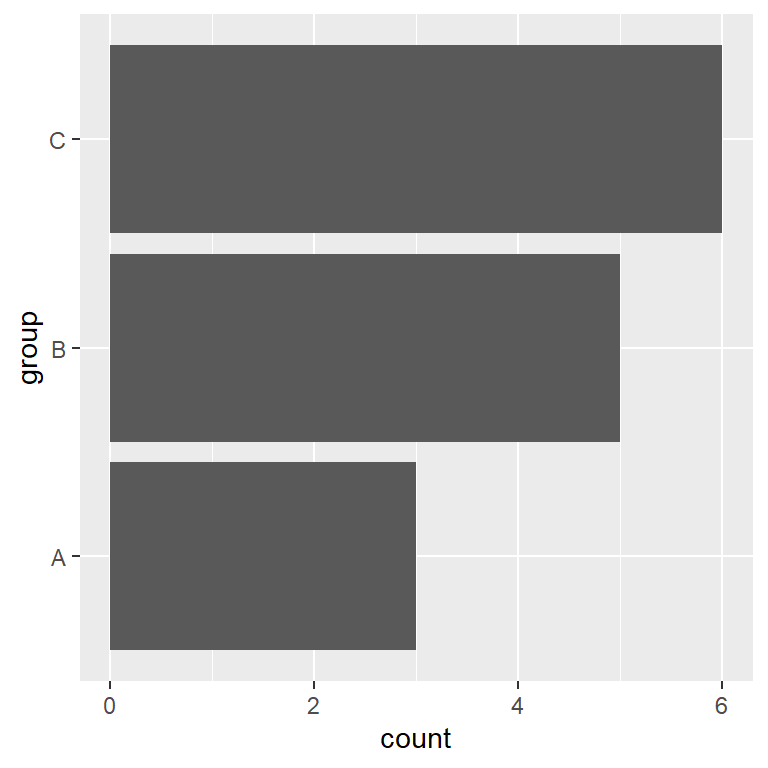Option 2: changing the `aes` variables order

``````# install.packages("ggplot2")
library(ggplot2)

ggplot(df, aes(x = count, y = group)) +
geom_bar(stat = "identity")``````## Order of the bars of the bar graph

The default order of the bars depend on the levels of the factor variable. In our example you can check the order of the bars with `levels(as.factor(df\$group))`. However, you reorder the bars in several ways: changing the limits with `scale_x_discrete`, modifying the levels order with `factor` or even using the `reorder` function.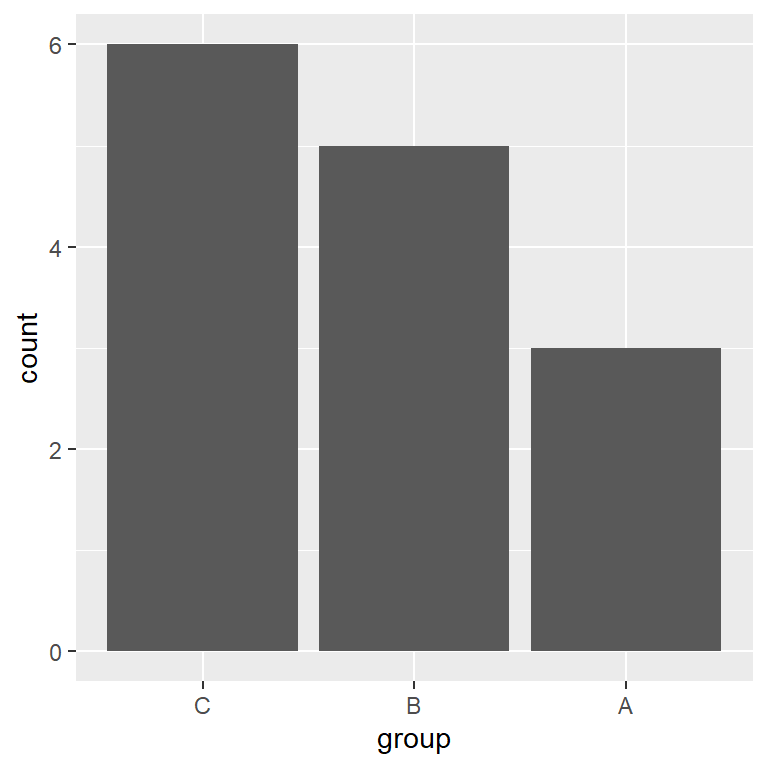``````# install.packages("ggplot2")
library(ggplot2)

ggplot(df, aes(x = group, y = count)) +
geom_bar(stat = "identity") +
scale_x_discrete(limits = c("C", "B", "A"))

# Or changing the levels of the factor variable
ggplot(df, aes(x = factor(group, levels = c("C", "B", "A")), y = count)) +
geom_bar(stat = "identity")``````

## Adding labels to the bars

In some scenarios its interesting to add labels to display the count for each bar or any text describing the bars. For that purpose, you can use the `geom_text` (or `geom_label`) function and set the labels inside the `label` argument of `aes` and changing its vertical alignment with `vjust`.

Labels inside the bars

``````# install.packages("ggplot2")
library(ggplot2)

ggplot(df, aes(x = group, y = count)) +
geom_bar(stat = "identity") +
geom_text(aes(label = count), vjust = 2, colour = "white")

# Note that if you data is categorical
# you can show the count for each group with ..count..
ggplot(df2, aes(x = cat)) +
geom_bar() +
geom_text(aes(label = ..count..), stat = "count", vjust = 2, colour = "white")``````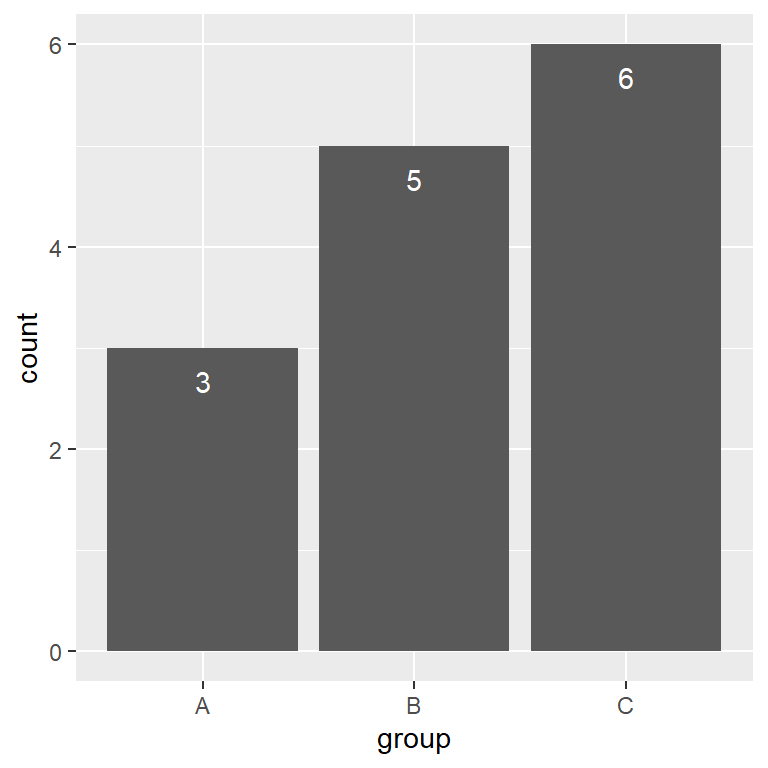Labels over the bars

``````# install.packages("ggplot2")
library(ggplot2)

ggplot(df, aes(x = group, y = count)) +
geom_bar(stat = "identity") +
geom_text(aes(label = count), vjust = -1, colour = "black") +
ylim(c(0, 6.5)) # Increase the limits of the Y-axis if needed``````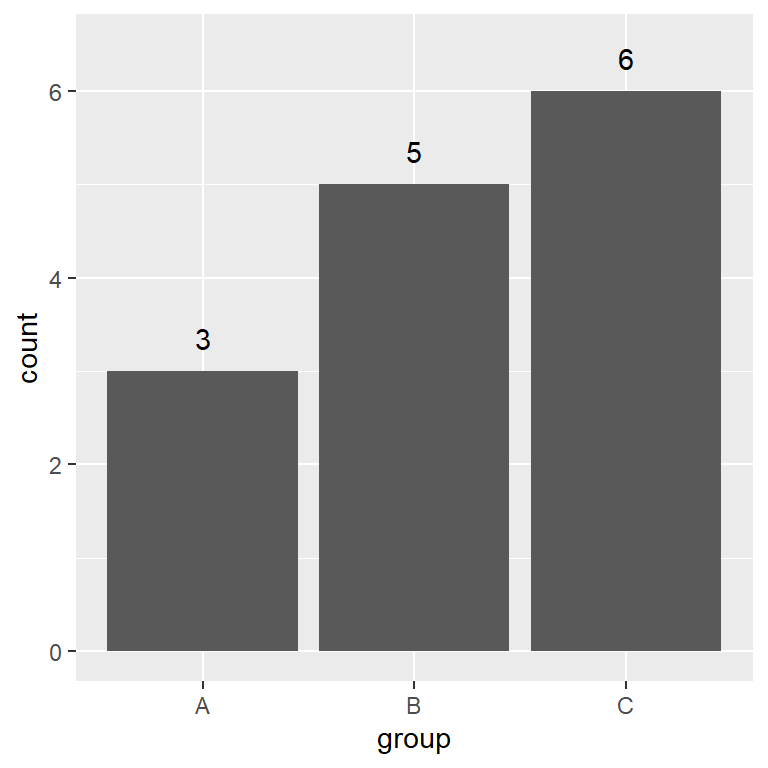## Color customization of the bars

It is possible to change the fill and the border color of the bars. You can set a color for all the bars, a color for each group or set the custom colors you want.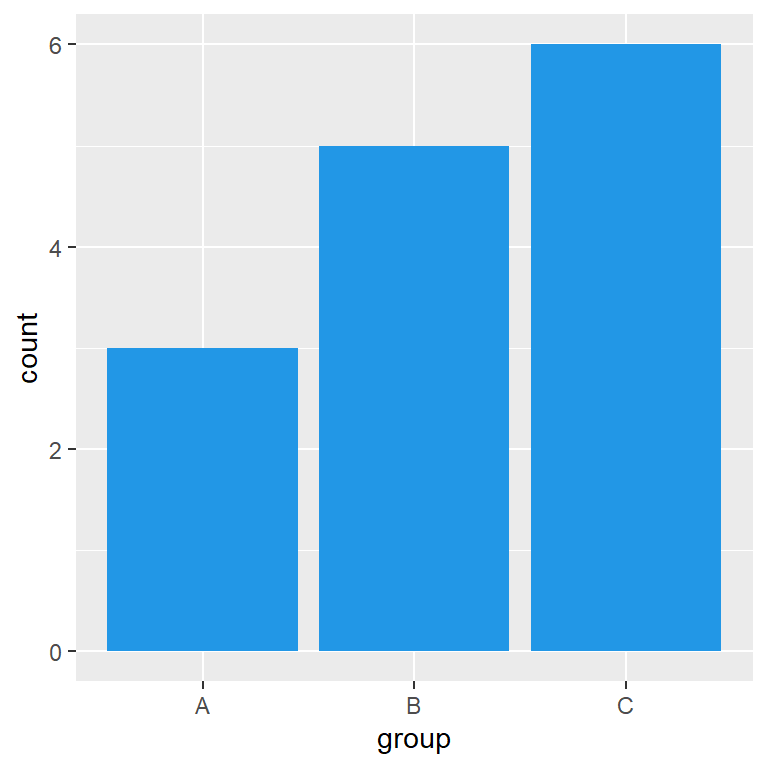Same color for all bars

By default, all the bars of the graph are gray. You can change this color passing a new color to the `fill` argument of `geom_bar`.

``````# install.packages("ggplot2")
library(ggplot2)

ggplot(df, aes(x = group, y = count)) +
geom_bar(stat = "identity", fill = 4)``````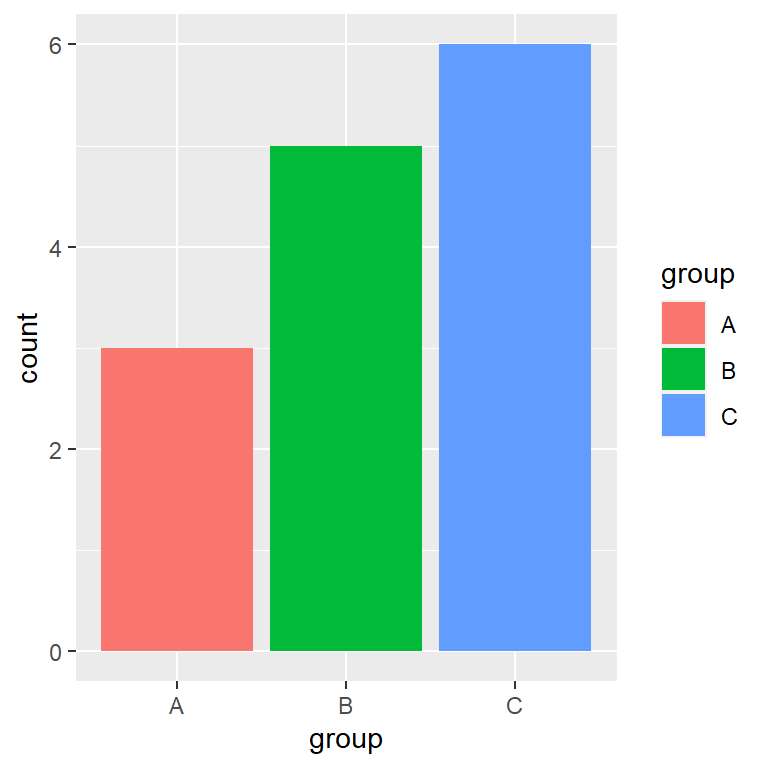Color by group

Note that you can also set a color for each group of your data. In this scenario, you will need to pass your categorical variable to `fill` but inside the `aes` function.

``````# install.packages("ggplot2")
library(ggplot2)

ggplot(df, aes(x = group, y = count, fill = group)) +
geom_bar(stat = "identity")``````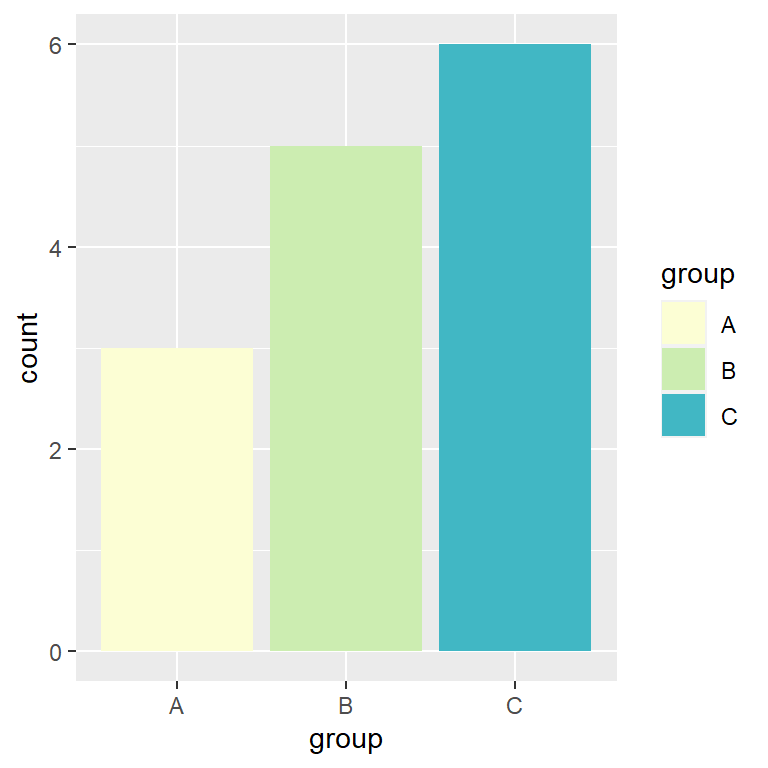Specify a color for each bar

When filling the colors of the bars by group the colors will be the ones of the default color palette from ggplot2. In order to override these colors you can specify them using other palette or with the `scale_fill_manual` function, both with an ordered vector of colors or with a named vector, where the names are the group labels.

``````# install.packages("ggplot2")
library(ggplot2)

ggplot(df, aes(x = group, y = count, fill = group)) +
geom_bar(stat = "identity") +
scale_fill_manual(values = c("#FCFED4", "#CCEDB1", "#41B7C4"))

# Or use a named vector with the group labels
ggplot(df, aes(x = group, y = count, fill = group)) +
geom_bar(stat = "identity") +
scale_fill_manual(values = c("A" = "#FCFED4", "B" = "#CCEDB1", "C" = "#41B7C4"))``````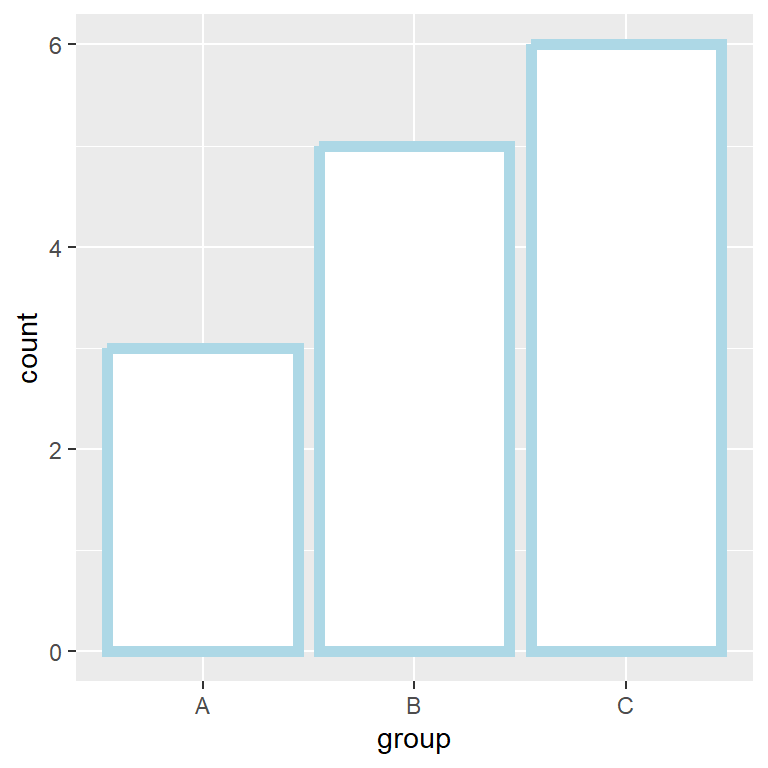Border color

If you use `color` instead of `fill` you can change the border color of the bars the same way you did with the fill color. In addition, you can change the line width and the line type with `lwd` and `linetype`, respectively.

``````# install.packages("ggplot2")
library(ggplot2)

ggplot(df, aes(x = group, y = count)) +
geom_bar(stat = "identity",
color = "lightblue",
lwd = 2, fill = "white")``````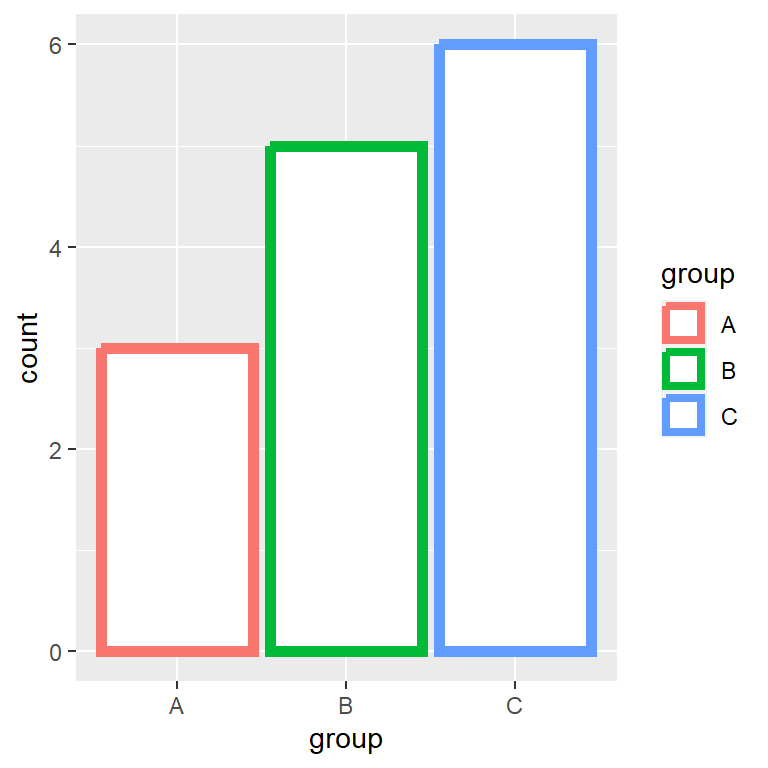Border color by group

In case you want to change the color by group pass the categorical variable to the `color` argument inside `aes`. Note that if you want to customize the colors you can use the `scale_color_manual` function.

``````# install.packages("ggplot2")
library(ggplot2)

ggplot(df, aes(x = group, y = count, color = group)) +
geom_bar(stat = "identity", lwd = 2, fill = "white")``````

## Legend customization

Legend position

The legend is positioned to the right by default, but you can change it with the `legend.position` argument of the `theme` function. Possible values are `"right"` (default), `"bottom"`, `"left"`, `"top"` and `"none"` to remove the legend of the bar plot.

``````# install.packages("ggplot2")
library(ggplot2)

ggplot(df, aes(x = group, y = count, fill = group)) +
geom_bar(stat = "identity") +
theme(legend.position = "bottom")``````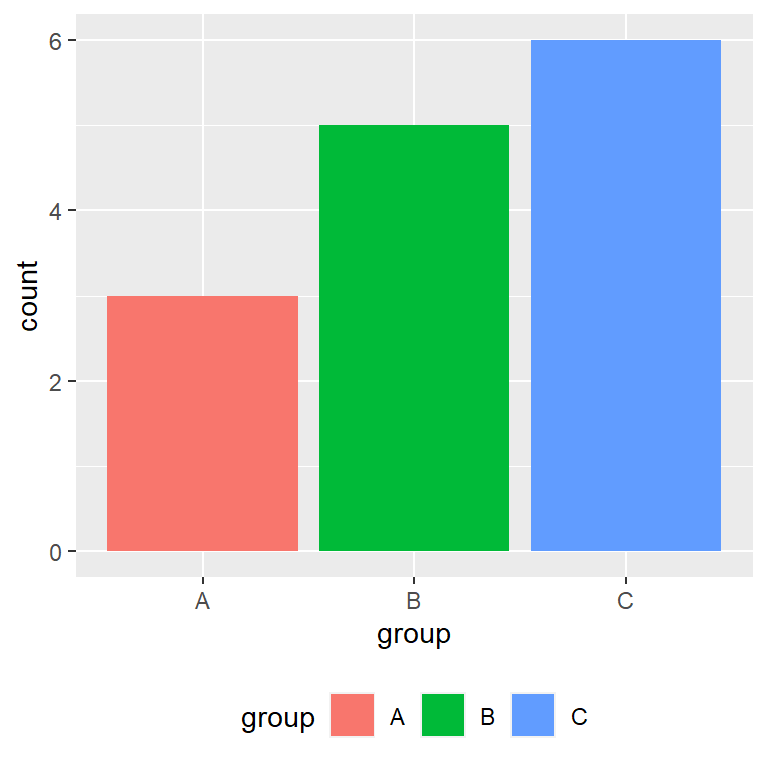Legend title

The default title of the legend is the name of the categorical variable. To override this name you can use the following line of code:

``````# install.packages("ggplot2")
library(ggplot2)

ggplot(df, aes(x = group, y = count, fill = group)) +
geom_bar(stat = "identity") +
guides(fill = guide_legend(title = "Legend title"))``````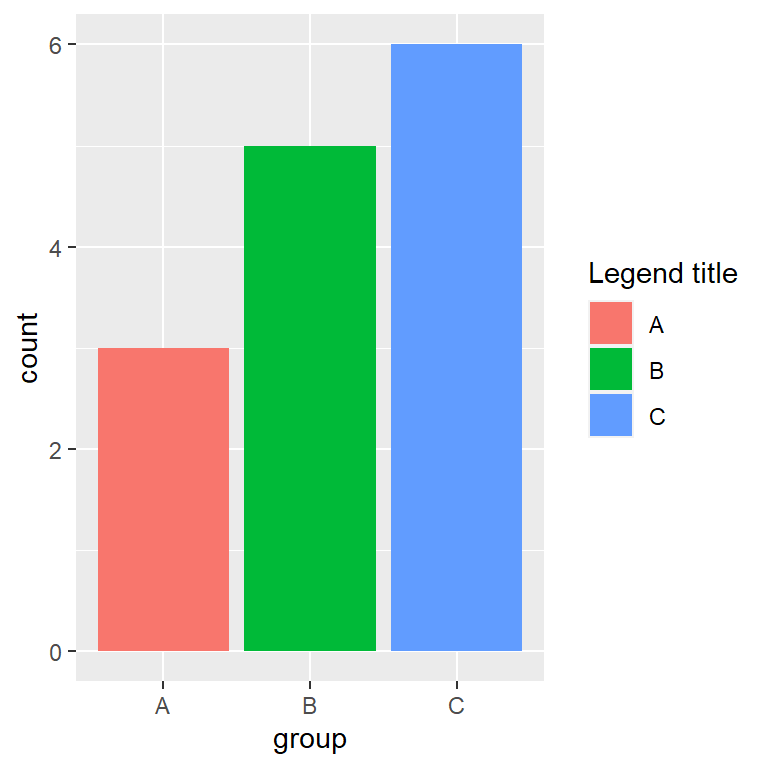Legend labels

Finally, if you want to set custom names for the legend change the levels of the categorical variable or use the `labels` argument of `scale_fill_hue` as follows.

``````# install.packages("ggplot2")
library(ggplot2)

ggplot(df, aes(x = group, y = count, fill = group)) +
geom_bar(stat = "identity") +
scale_fill_hue(labels = c("Group A", "Group B", "Group C"))``````